# CIE A Level Maths: Pure 3复习笔记8.2.1 Argand Diagrams - Basics

### Argand Diagrams - Basics

#### What is an Argand diagram?

• An Argand diagram is a geometrical way to represent complex numbers as either a point or a vector in two-dimensional space
• We can represent the complex number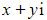by the point with cartesian coordinate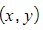• The real component is represented by points on the x-axis, called the real axis, Re
• The imaginary component is represented by points on the y-axis, called the imaginary axis, Im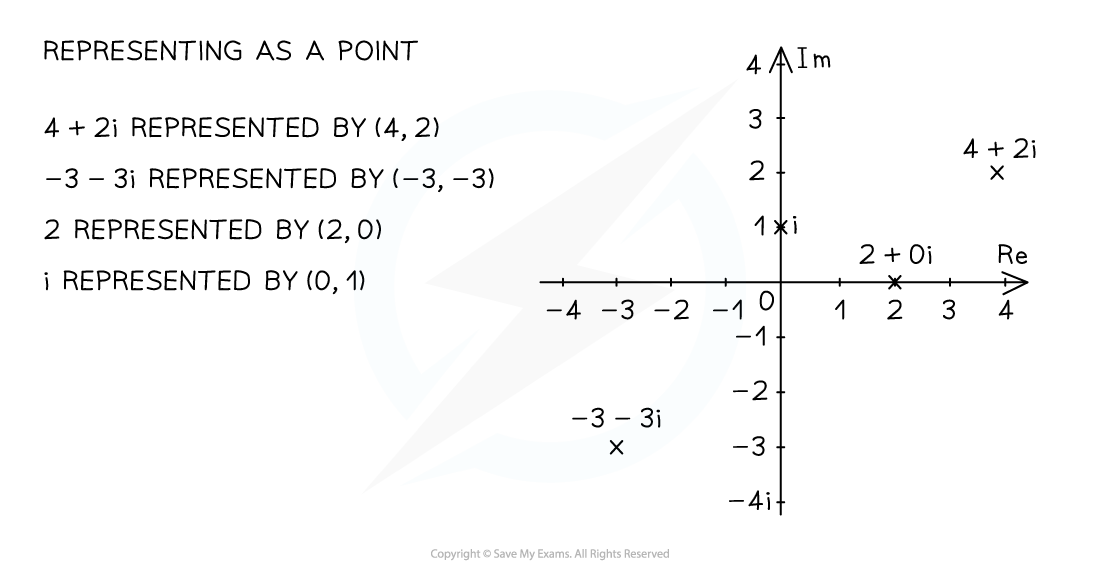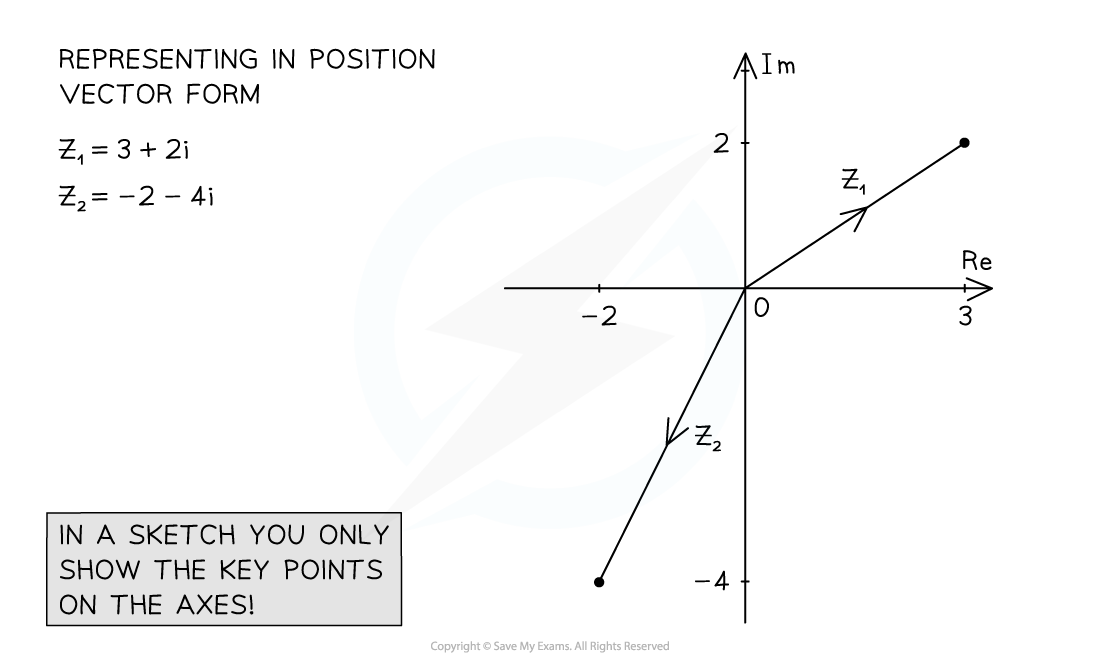• You may be asked to show roots of an equation in an Argan diagram
• First solve the equation
• Draw a quick sketch, only adding essential information to the axes
• Plot the points and label clearly

#### How can I use an Argand diagram to visualise |z1 + z2| and |z1 - z2|?

• Plot two complex numbers z1 and z2
• Draw a line from the origin to each complex number
• Form a parallelogram using the two lines as two adjacent sides
• The modulus of their sum |z1 + z2| will be the length of the diagonal of the parallelogram starting at the origin
• The modulus of their difference |z1 - z2| will be the length of the diagonal between the two complex numbers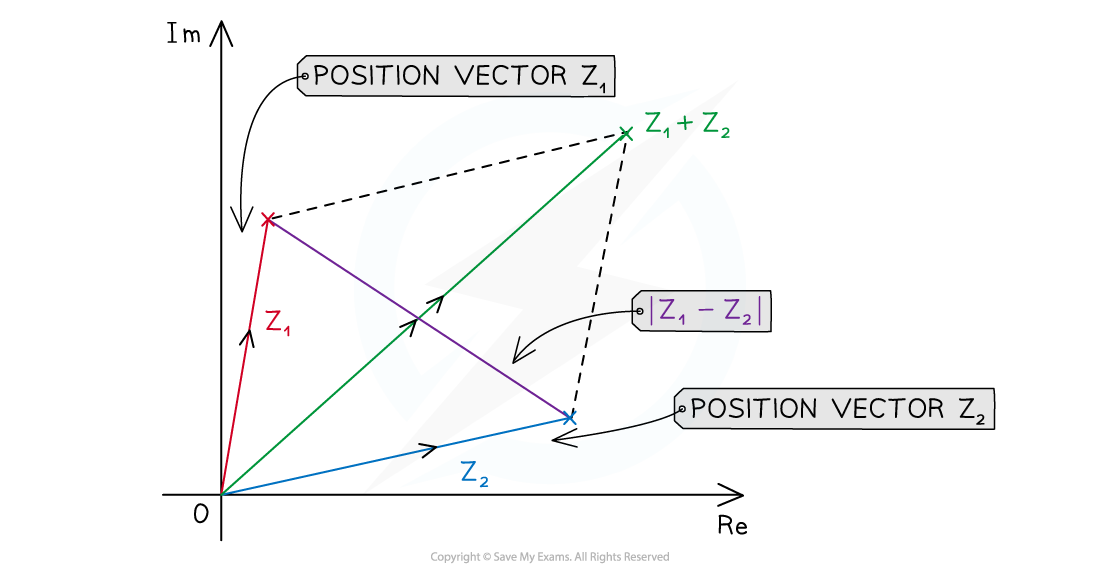#### Worked Example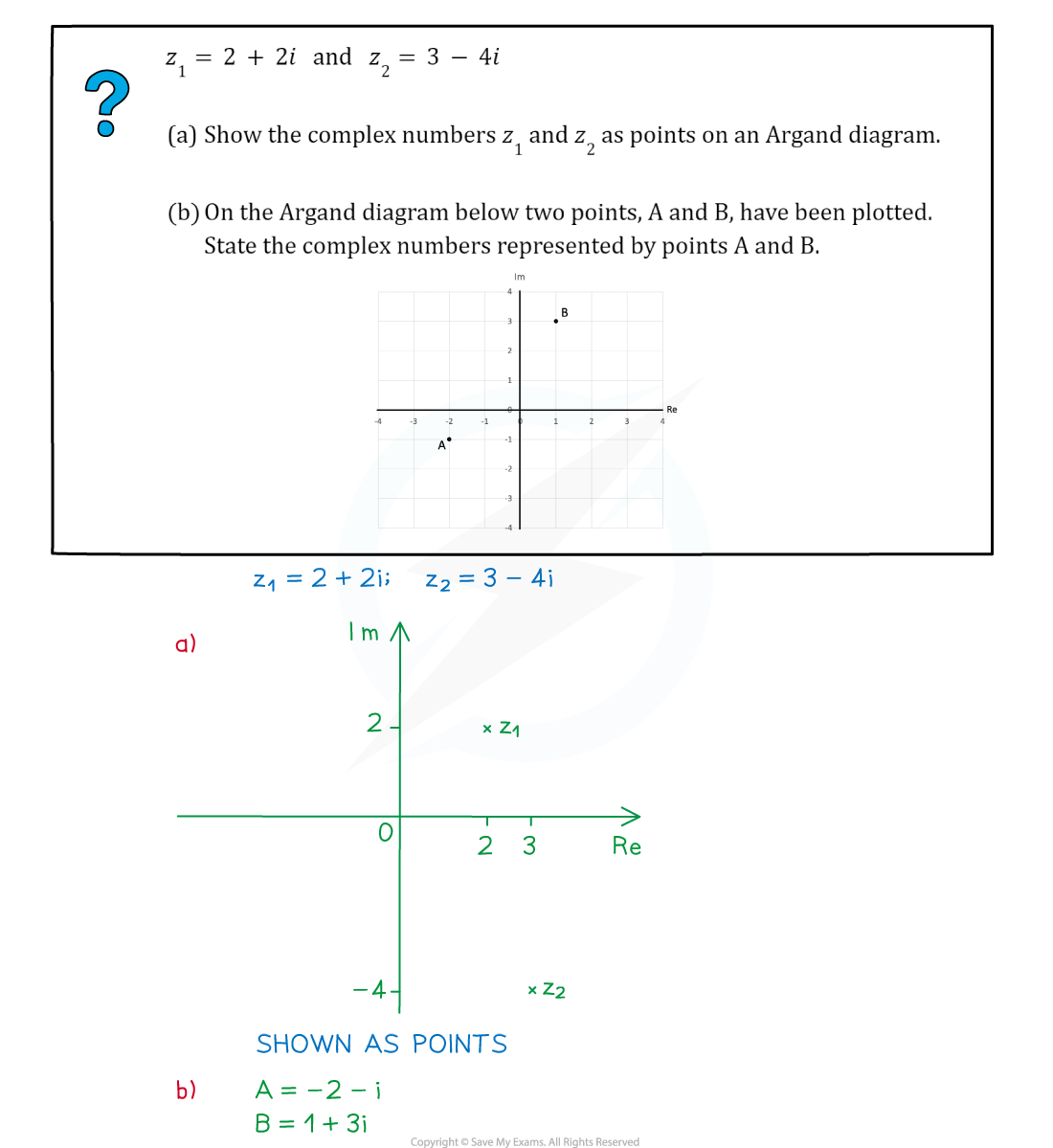#### Exam Tip

• When setting up an Argand diagram you do not need to draw a fully scaled axes, you only need the essential information for the points you want to show, this will save a lot of time.